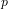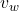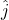An airplane flies with an unknown velocity due east. The plane is experiencing wind of 12 m/s due north which produces a resulta

Question

An airplane flies with an unknown velocity due east. The plane
is experiencing wind of 12 m/s due north which produces a
resultant velocity of 35.11 m/s northeast. Determine the
unknown velocity produced by the plane engines.

in progress 0
6 months 2021-07-15T19:30:46+00:00 2 Answers 20 views 0

The unknown velocity produced by the planes engines is approximately 33 m/s due east

Explanation:

The given parameters are;

The direction of the airplane’s flight = East ward

The speed of the wind experienced by the plane = 12 m/s

The direction of the wind = Due north

The resultant velocity of the = 35.11 m/s northeast

Let the velocity of the plane be vWe present the velocity of the plane and the velocity of the wind vectorially as follows;

Velocity of the plane = v·The velocity of the wind =·= 12·The resultant velocity, vof the airplane is given by the following relation from Pythagoras’s theorem for right triangles;

v= √((v)² + ()²)

Therefore;

35.11 = √((v)² + (12)²)

v= √(35.11² – 12²) = 32.9956375904 ≈ 33 m/s

Therefore;

The velocity of the airplane = 33 m/s due east

The unknown velocity produced by the planes engines ≈ 33 m/s due east

32.99m/s

Explanation:

Using the formula for calculating the resultant velocity according to Pythagoras theorem

R² = 12²+x²

35.11² = 12²+x²

X² = 35.11²-12²

X² = 1232.7121-144

X² = 1088.8121

X = √1088.8121

X = 32.99m/s

Hence the unknown velocity produced by the plane engines is 32.99m/s